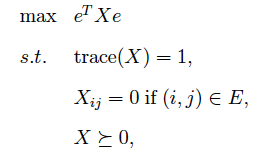# Free tool to use for this SDP?

 1 I've looked into cvxopt and I don't immediately see a way to turn this into problem formulation they support. Is there a free tool I can use to solve SDP below?Update I got it working following Joachim's suggestion. Needed couple additional details (which are probably obvious to optimization experts), latexized write-up is here asked 02 Mar '11, 04:01Yaroslav Bul... 43●1●6 accept rate: 0%

 3 Your problem is specified in the dual form according to CVXOPT's convention. If you write your problem as max. -Tr(Z, e*e') s.t. Tr(Z, I) = 1 Tr(Z, ei*ej') = 0, for all (i,j)in E Z >= 0  you are very close to specifying the dual problem in CVXOPT. You only have a single semidefinite variable (N=1). so specify G^T = [vec(I)^T; vec(e_i1 * e_j1^T)^T; ... ; vec(e_ip * e_jp^T)^T] in which case you get the problem max. -z'*vec(e*e') s.t. G^T * z = (1, 0, ..., 0)^T z >= 0.  where z := vec(Z). The later is vectorized form used by most SDP solvers (SeDuMi, SDTP3,...) You will only be able to solve very moderate size instances using a primal-dual algorithm. If your graph happens to be chordal (or almost chordal), you can solve much larger problems using either a primal or dual method based chordal matrix completion. The following packages implement those ideas and are plugins for CVXOPT: http://abel.ee.ucla.edu/chompack/ http://abel.ee.ucla.edu/smcp/ answered 02 Mar '11, 11:01Joachim Dahl 116●3 accept rate: 33% thanks, I got it working now (03 Mar '11, 08:24) Yaroslav Bul...
 1 Wouldn't this work? dim(s_0) = 0 G_1 = -id h_1 = 0  (this sets x to the entries of s_1) c = -e (e in R^(n^2))  express the two constraints in the matrix A (I suppose E is some fixed set?) answered 02 Mar '11, 07:04Hans 109●3 accept rate: 0%
 toggle preview community wiki

By Email:

Markdown Basics

• *italic* or _italic_
• **bold** or __bold__
• image?![alt text](/path/img.jpg "Title")
• numbered list: 1. Foo 2. Bar
• to add a line break simply add two spaces to where you would like the new line to be.
• basic HTML tags are also supported

Tags:

×190